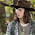## Monday, July 23, 2018

Eurocode 5 deals with the limit state design of timber structures. In this post, we are going to present an example on how we can design axially loaded timber columns according to the requirements of Eurocode 5.

Example
Verify the capacity of 200 mm x 100 mm timber section of strength class C18 to satisfy ultimate limit state requirements under the following conditions:

Service Condition: Class 2
Characteristic permanent action Gk = 10 kN
Variable medium term action = 25 kN
Height of column = 3.0m
Support condition: Effectively held in position but nor in direction in both axes.

Solution
Geometric properties
Depth of member h = 200 mm
Width of member b = 100 mm

Area of member  A =  bh = 200 x 100 = 20000 mm2
Second moment of area about the y-y axis Iy = bh3/12 = (100 x 2003)/12 = 6.667 x 107 mm4
Radius of gyration about the y-y axis i= sqrt(Iy/A) = sqrt(6.667 x 107/20000 ) = 57.74 mm

Second moment of area about the z-z axis Iz = hb3/12 = (200 x 1003)/12 = 1.667 x 107 mm4
Radius of gyration about the z-z axis i= sqrt(Iz/A) = sqrt(1.667 x 107/20000 ) = 28.86 mm

Effective length about the y-y axis LE,y = Effective length about the z-z axis LE,z = 1.0 L = 3000 mm

Therefore slenderness ratio about the y-y axis;
λy = LE,y/iy =3000/57.74 = 51.957

The slenderness ratio about the z-z axis;
λz = LE,z/iz =3000/28.86 = 103.95

Timber Properties (Table 1 BS EN 338:2003(E))
For strength class C18;
Characteristic compression strength parallel to the grain fc.0.k = 18 N/mm2

Fifth percentile modulus of the elasticity parallel to the grain E0.05 = 6.0 kN/mm2

Partial safety factors
UKNA to BS EN 1990:2002, Table NA.A1.2(B)) for the ULS
Permanent actions, γG = 1.35
Variable actions, γQ = 1.5

Material factor for solid timber, γM = 1.3 (UKNA to EC5, Table NA.3)

Design Action
Design Action at ultimate limit state  NEd = 1.35Gk + 1.5Qk
NEd = 1.35(10) + 1.5(25) = 51 kN = 51000 N

Modification factors
Factor for medium duration loading and service class 2, kmod.med = 0.8 (EC5, Table 3.1)
System strength factor, (not relevant) ksys = 1.0

Therefore, the design compression stress αc,0,d = NEd/A = 51000/20000 = 2.55 N/mm2

The design compression strength fc,0,d = (kmod.med ksys fc.0.k)/ γ
fc,0,d = (0.8 x 1.0 x 18)/1.3 = 11.08 N/mm2

Buckling Resistance (clause 6.3.2 EC5)
Relative slenderness about the y-y axis
λrel,y = (λy /π) x sqrt(fc.0.k/E0.05) = (51.957/π) x sqrt(18/6000) = 0.9058 (equation 6.21 EC5)

Relative slenderness about the z-z axis
λrel,z = (λz /π) x sqrt(fc.0.k/E0.05) = (103.95/π) x sqrt(18/6000) = 1.81 (equation 6.21 EC5)

As both relative slenderness ratios are greater than 0.3, the conditions in clause 6.3.2(3):

Maximum relative slenderness ratio of the column = λrel,z = 1.81

Factor βc for solid timber βc = 0.2 (EC5, equation (6.29))

The factor kz (equation 6.28 EC5)

kz = 0.5[1 + 0.2(1.81 - 0.3) + 1.812] = 2.289

Instability factor (equation 6.26 EC5)
kzc = 1/[2.289 + sqrt(2.2892 - 1.812)] = 0.27

Design buckling strength kzc .fc,0,d = 0.27 x 11.08 = 2.9916 N/mm2

Therefore, design stress/design buckling strength ratio = 2.55/2.9916 = 0.852 < 1.0 Ok

Therefore, the section is satisfactory.

1.2.